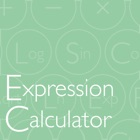Expression Calculator

• SEARCH TYPE

All iPhone applications categories

All iPhone games categories# Expression Calculator

by: Orela.org 0 0

## Screenshots

Description

"Expression Calculator" is a scientific calculator features at a simple and stylish.
It can edit a formula that you enter, and you are possible to copy and paste.

In addition, if the input to Equation, difficult also calculated forte.
Let's try to calculate the following formula using existing calculator.

"6 + 8 × 0 ÷ 2"

The answer is "6".

When work with a calculator, did confusion in priorities?
If it is "Expression Calculator", you can answer just be entered as an expression.

[Feature List]
•Arithmetic
•Calculation the brackets
•Editing of the formula
•Summarize each line results
•Various function
•Copy of expressions and calculation results